﻿ C++ Exercises: Check whether a number is Lychrel number or not - w3resource

# C++ Exercises: Check whether a number is Lychrel number or not

## C++ Numbers: Exercise-11 with Solution

Write a program in C++ to check whether a number is a Lychrel number or not.

Pictorial Presentation: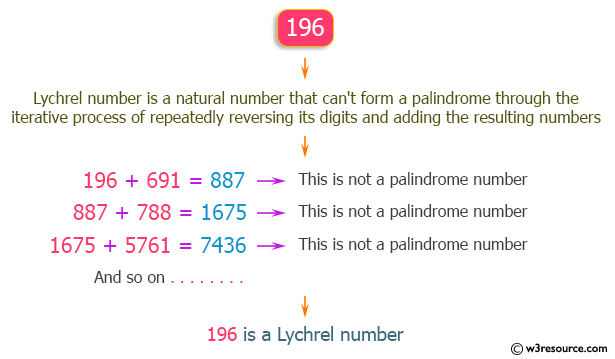Sample Solution:

C++ Code :

``````#include <iostream>
using namespace std;

long long int numReverse(long long int number)
{
long long int rem = 0;
while (number > 0)
{
rem = (rem * 10) + (number % 10);
number = number / 10;
}
return rem;
}
bool is_Palindrome(long long int num)
{
return (num == numReverse(num));
}
bool isLychrel(int num, const int iterCount = 500)
{
long long int temp = num;
long long int rev;
for (int i = 0; i < iterCount; i++)
{
rev = numReverse(temp);
if (is_Palindrome(rev + temp))
return false;
temp = temp + rev;
}
return true;
}
int main()
{
int lyno;
bool l;
cout << "\n\n Check whether a given number is a Lychrel number: \n";
cout << " ------------------------------------------------------\n";
cout << " Input a number: ";
cin >> lyno;
l = isLychrel(lyno);
if (l==0)
{
cout <<" The number is not a Lychrel number."<<endl;
}
else
if(l==1)
{
cout<<" The number is a Lychrel number."<<endl;;
}
return 0;
}
``````

Sample Output:

```Check whether a given number is a Lychrel number:
------------------------------------------------------
Input a number: 196
The number is a Lychrel number.
```

Flowchart: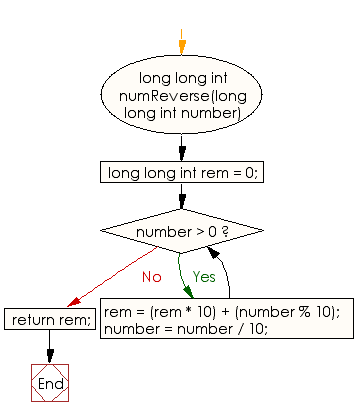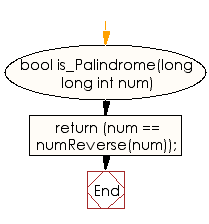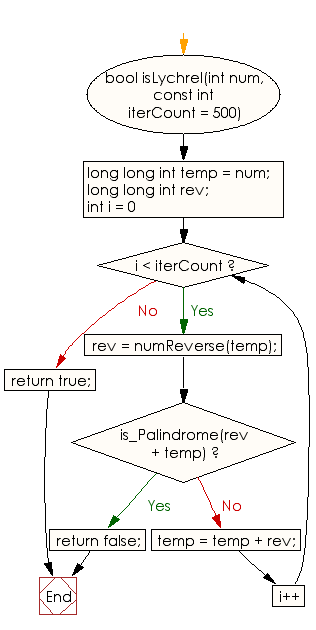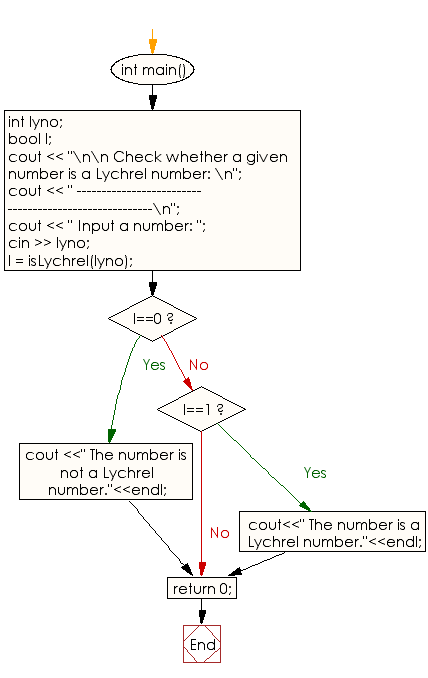C++ Code Editor: